Updated date:

# Excel Number Format Problem

I love giving tips and advice on how to use various computer programs.

A common and frustrating problem when working in Excel is when you encounter a spreadsheet which has number formats that are not recognised as numbers. Usually this occurs where the columns are being read as text formats and cannot be converted to number formats. This is a common problem when numbers are in the European number format with the comma “,” being used as a decimal separator and a full stop used as a thousands seperator.

This article explains how to fix this number formatting problem in Excel and reformat numbers into a format which does work.

## The Problem: Numbers Are Not Recognised by Excel

The below screenshot shows the problem. Column C is in a European number format which is not recognized by U.S. versions of Excel. No matter how I format column C, I can’t get it into a format which is recognized as numbers. If I click on any of these cells and try to reformat them as numbers in Excel, it does not work. The cells are in a format which cannot be recognized by Excel as numbers.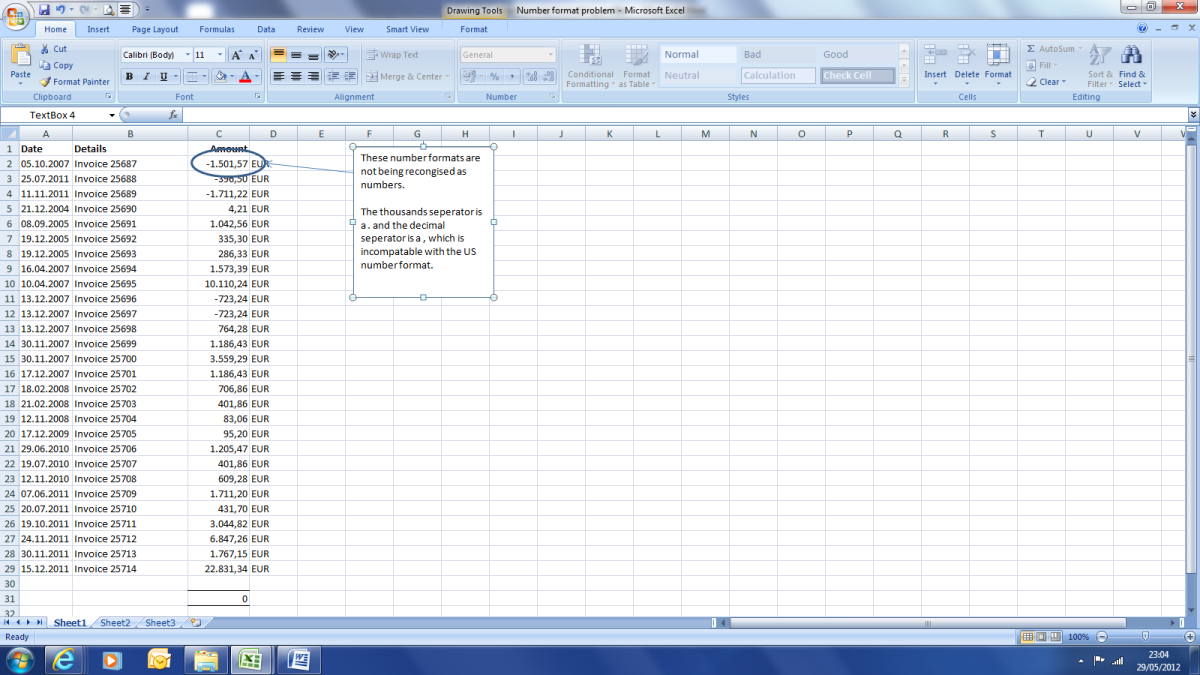Column C is in a European number format. This is not recognised as numbers by my version of excel

## Step 1: Highlight Column and Remove the Thousands Separator

The first thing we want to do is remove the decimal point ‘.’ as a thousand separator. To do this, highlight the column with the numbers and click on find-replace. Find the decimal ‘.’ and replace it with nothing (i.e., don’t write any character in the replace box).

When we have performed this find and replace it will remove the . from the numbers, and we have a number format without the . thousands separator. This fixes half of the Excel number problem.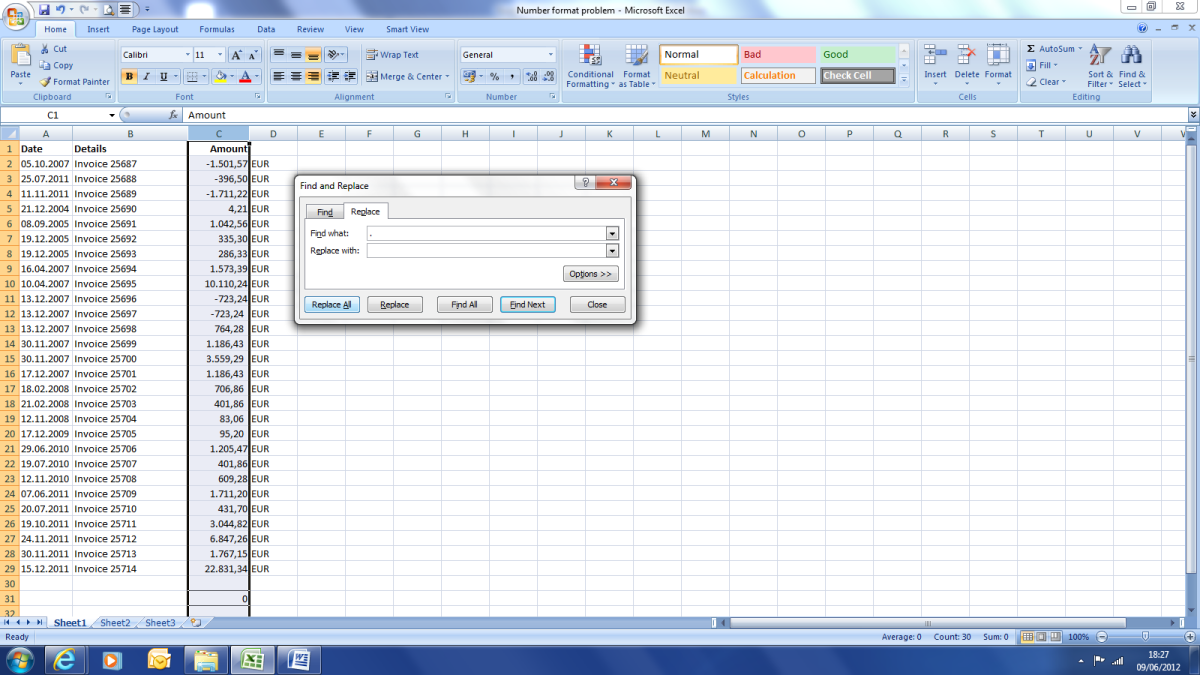Use the find and replace function to find the thousands decimal and remove it.

## Step 2: Remove the Comma (,) as the Decimal Separator

European number formats use a comma (,) for a decimal place. This is not recognised by the US version of Excel. What we need to do is remove the comma and replace it with a decimal place (.) To do this, we use the find and replace function again to highlight column C and find the comma, and replace it with a full stop (.)

Once we have completed this step, the numbers will now show a . instead of a comma, for the decimal separator.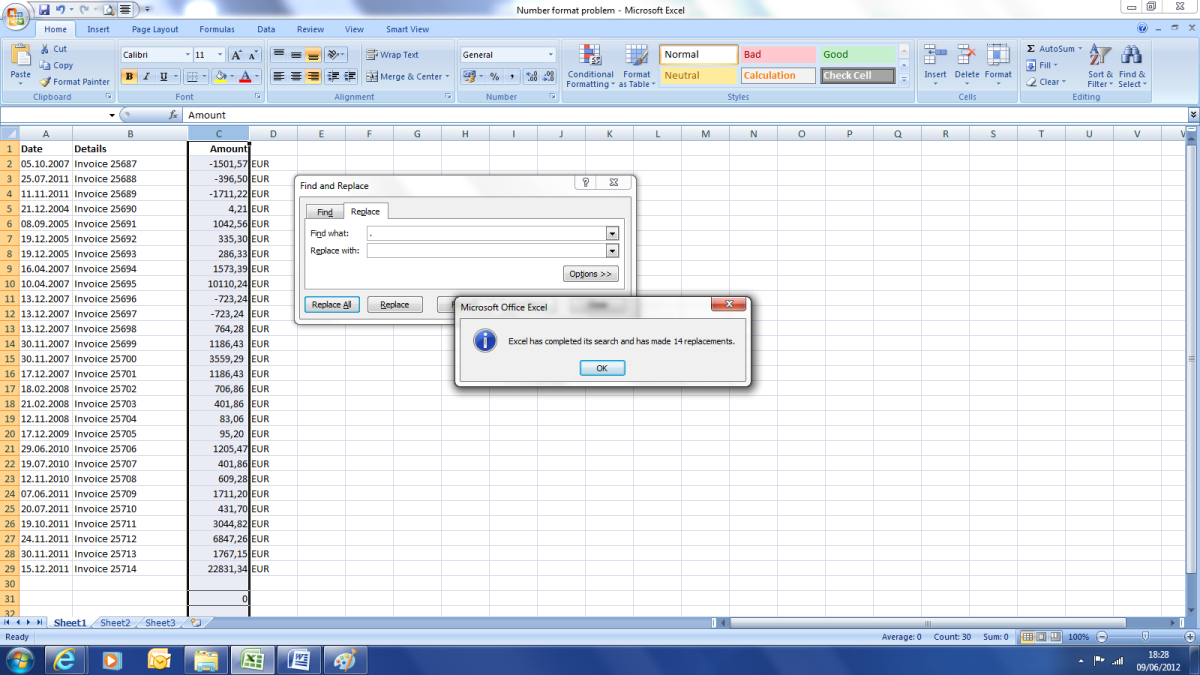After the find and replace function we can see the . has been removed.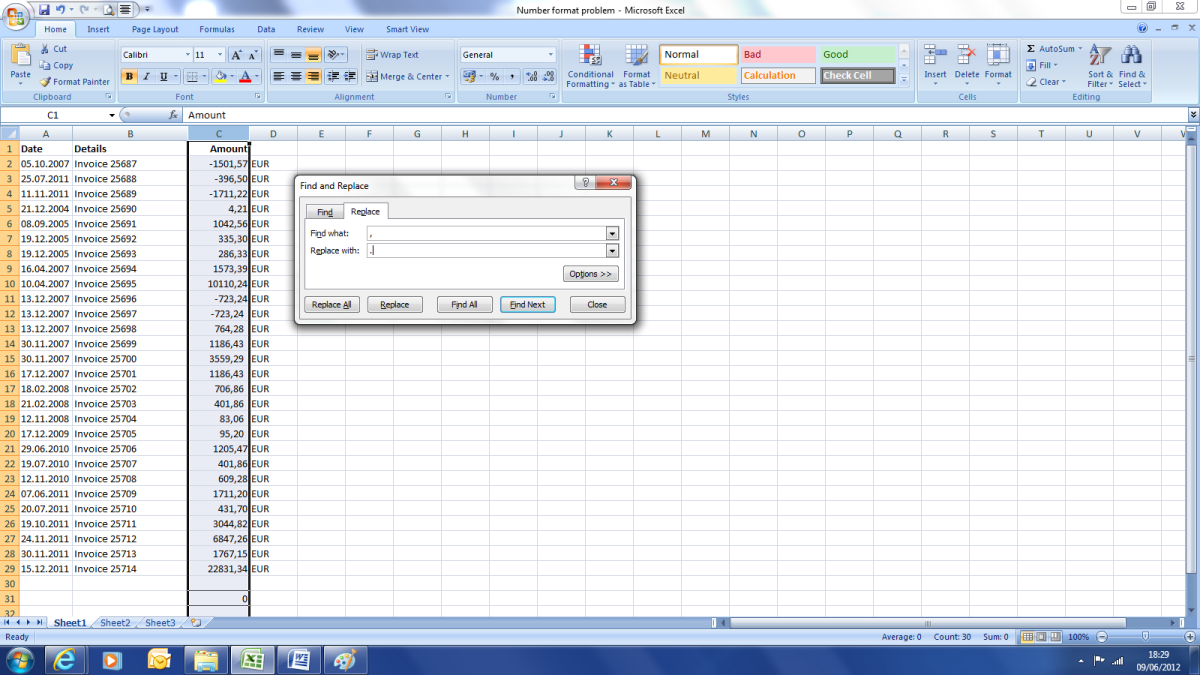Search on the , decimal seperator and replace it with a .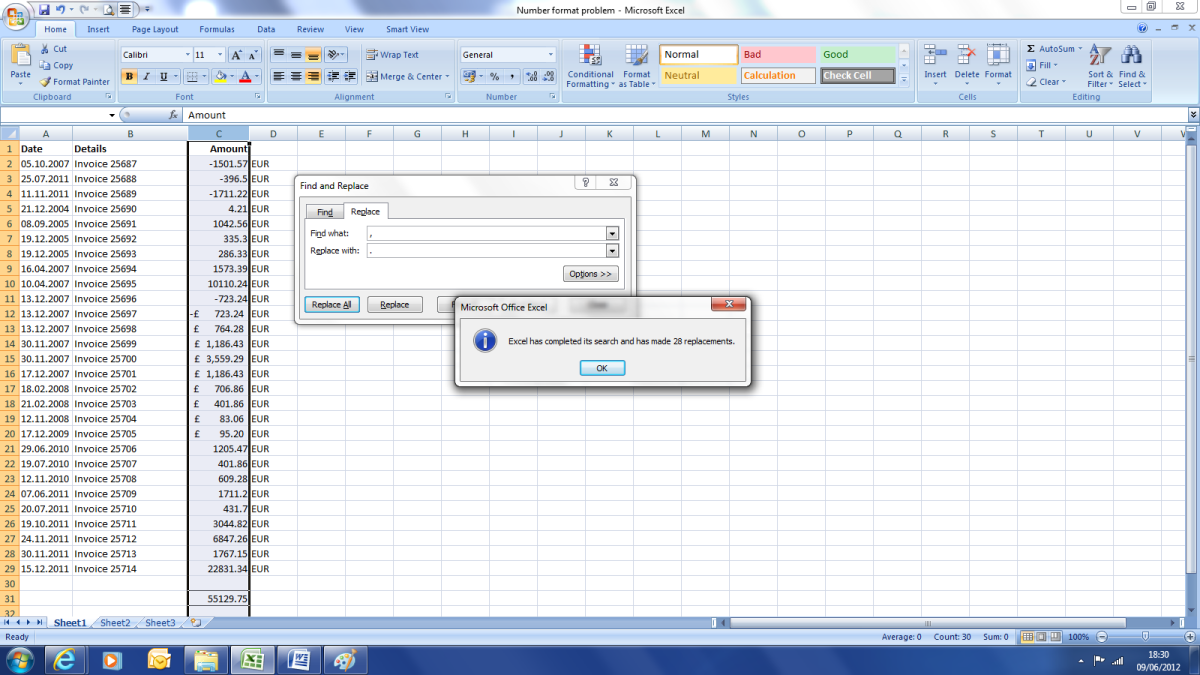Once we have run the find and replace function we now have a . as a decimal seperator which is recognised by excel.

## Excel Number Problem Fixed

As we can now see, after using find and replace to remove the problematic thousands and decimal separators, we have numbers in a format which is recognised by Excel and can be summed.

## More Excel Problems Solved

• Excel problems - Fix date formats
One of the most frustrating problems in Excel is having a spreadsheet which dates are in a funny format that isn’t recognised by Excel. This hub explains how to fix this problem and put these troublesome dates into a format that Excel will recognise.